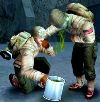###Author Topic: Dirty Bomb - (Another) spiritual successor to W:ET  (Read 9400 times)

#### Kissaki

• Forum God
••• Posts: 10.338##### Dirty Bomb - (Another) spiritual successor to W:ET
« on: 04.06.2015, 20:25:57 »
Splash Damage, developer of the original Wolfenstein: Enemy Territory, as well as the two spiritual successors Enemy Territory: Quake Wars and Brink,
released the free-to-play, open beta of Dirty Bomb on steam.

It does feel a bit like W:ET.

It’s fun.
But I’m sceptical about the pay-to-win model; you unlock avatar upgrades randomly, with 3 stat boosters of increasing power.

Anyway, try it out!What do you think? Do you enjoy it?

ヤン

#### Gauki

• Forum God
••• Posts: 2.110##### Re: Dirty Bomb - (Another) spiritual successor to W:ET
« Reply #1 on: 07.06.2015, 23:21:28 »
It’s fun.
But I’m sceptical about the pay-to-win model; you unlock avatar upgrades randomly, with 3 stat boosters of increasing power.

what/why u have to pay for sometiing in a free to play game? O.o

#### Kissaki

• Forum God
••• Posts: 10.338##### Re: Dirty Bomb - (Another) spiritual successor to W:ET
« Reply #2 on: 09.06.2015, 19:40:01 »
It’s a typical free to play model. You *can* buy stuff you can otherwise unlock through playtime.

I didn’t feel those stats unfainess yet, so I guess the model is not too bad.
« Last Edit: 09.06.2015, 22:26:18 by Kissaki »

ヤン

#### Kissaki

• Forum God
••• Posts: 10.338##### Re: Dirty Bomb - (Another) spiritual successor to W:ET
« Reply #3 on: 21.06.2015, 18:21:37 »
If you want to adjust your HUD (e.g. put healthbar, ammo, ability cooldown to the middle, near the cursor)
http://wezelkrozum.com/temp/xt_hudconfig/v3/

Generate, and put into bottom of
Code: [Select]
`C:\Users\<user>\Documents\My Games\UnrealEngine3\ShooterGame\Config\ShooterUI.ini`
« Last Edit: 21.06.2015, 22:12:33 by Kissaki »

ヤン

#### FuckBird##### Re: Dirty Bomb - (Another) spiritual successor to W:ET
« Reply #4 on: 21.06.2015, 21:40:32 »
WUP WUP New ET

#### Kissaki

• Forum God
••• Posts: 10.338##### Re: Dirty Bomb - (Another) spiritual successor to W:ET
« Reply #5 on: 21.06.2015, 21:56:36 »
A centered UI
Code: [Select]
`[ShooterGame.SGUIHUDPlayer]NativeHUDResolution=(X=1280, Y=720)ScopeInfoPosition=(Attach=(H=HA_CENTER), PixelOffset=(Y=500)) ConsoleMessageLogPosition=(Align=(H=HA_right, V=HA_BOTTOM), Attach=(H=HA_RIGHT, V=HA_TOP, VA="ChatInput"), Skew=0)ChatInputPosition=(Align=(H=HA_RIGHT, V=HA_BOTTOM), Attach=(H=HA_RIGHT, V=HA_BOTTOM,VA="Minimap"), PixelOffset=(Y=-40), Skew=0)IncapMenuPosition=(Align=(H=HA_CENTER, V=HA_BOTTOM), Attach=(H=HA_CENTER, V=HA_TOP, VA="DeathInfoCard")) GameWaveTimerPosition=(Align=(H=HA_LEFT, V=HA_BOTTOM), Attach=(H=HA_LEFT, V=HA_BOTTOM), PixelOffset=(Y=35), Skew=0)ObjectiveProgressionPosition= (Align=(H=HA_LEFT, V=HA_BOTTOM), Attach=(H=HA_LEFT, V=HA_BOTTOM), PixelOffset=(Y=-10), Skew=0)ObjectiveClockPosition=ObjectiveProgressionNotificationPosition=ObjectivePosition=ExpCounterPosition=GameplayNotificationPosition= MinimapPosition=(Align=(H=HA_CENTER, V=HA_BOTTOM),Attach=(H=HA_CENTER, V=HA_BOTTOM), PixelOffset=(X=104, Y=20), Skew=0)WeaponAmmoPosition=(Attach=(H=HA_CENTER, V=HA_CENTER, VA="PlayerHealth"), PixelOffset=(X=15, Y=34), ShadowOffset=(X=1, Y=1), Skew=0)AbilityCooldownPosition=(Align=(V=HA_TOP), Attach=(H=HA_CENTER, V=HA_BOTTOM, VA="PlayerHealth"), PixelOffset=(X=-256, Y=-20), ShadowOffset=(X=1, Y=1), Skew=0)PlayerHealthPosition=(Align=(V=HA_BOTTOM), Attach=(H=HA_CENTER, V=HA_CENTER), PixelOffset=(X=-150, Y=225), ShadowOffset=(X=1, Y=1), Skew=0) ObituaryMessageLogPosition=(Attach=(H=HA_RIGHT,V=HA_TOP))InteractionFillbarPosition=(Attach=(H=HA_CENTER),PixelOffset=(Y=420))SubtitlesPosition=(Attach=(H=HA_CENTER, V=HA_BOTTOM), PixelOffset=(Y=710))BadgeNotificationPosition=(Attach=(H=HA_CENTER, V=HA_TOP), PixelOffset=(Y=50))ObjectiveNotificationPosition=(Attach=(H=HA_CENTER, V=HA_TOP, VA="BadgeNotification"), PixelOffset=(Y=150), ShadowOffset=(X=1, Y=1))GameInfoPosition=(Attach=(H=HA_CENTER, V=HA_TOP))SpectatorInfoPosition=(Attach=(H=HA_CENTER,V=HA_BOTTOM),PixelOffset=(Y=150), ShadowOffset=(X=-1, Y=-1))DetectedNotificationPosition=(Align=(H=HA_CENTER, V=HA_CENTER), Attach=(H=HA_CENTER, V=HA_BOTTOM), PixelOffset=(Y=100))ReadyUpPosition=(Align=(V=HA_TOP,H=HA_CENTER), Attach=(H=HA_CENTER, V=HA_TOP))DeathInfoCardPosition=(Align=(V=HA_BOTTOM,H=HA_CENTER), Attach=(H=HA_CENTER, V=HA_BOTTOM), PixelOffset=(Y=0))ActionMenuPosition=(Align=(H=HA_LEFT, V=HA_TOP), Attach=(H=HA_LEFT, V=HA_BOTTOM,VA="Objective"), PixelOffset=(Y=-2))CharacterSelectionPosition=(Align=(H=HA_CENTER), Attach=(H=HA_CENTER, V=HA_BOTTOM) PixelOffset=(X=0, Y=100))MaxNumHitReticules=16MaxNumConsoleMsg=10MaxConsoleMsgTime=-1MaxConsoleParentDisplayTime=8ConsoleMsgYBufferHeight=1MaxNumObituaryMsg=8MaxObituaryMsgTime=5ObituaryMsgYBufferHeight=1m_bEnableHudShake=false`from http://forums.dirtybomb.nexon.net/discussion/2134/centered-ui-shooterui-ini

ヤン

#### Kissaki

• Forum God
••• Posts: 10.338##### Re: Dirty Bomb - (Another) spiritual successor to W:ET
« Reply #6 on: 28.10.2015, 20:15:48 »
update trailer

ヤン

#### Gauki

• Forum God
••• Posts: 2.110##### Re: Dirty Bomb - (Another) spiritual successor to W:ET
« Reply #7 on: 09.11.2015, 23:58:55 »
looks nice ^^

#### Kissaki

••